##### The Japanese Bonsai specialist
Direct order Contact Help / Services Newsletter# Pulvérisateur berthoud 1 litre

› Bonsai tools › Watering equipment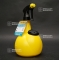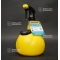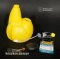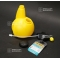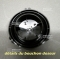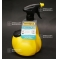ref. : 10061

8,40

Available quantity : 9Order

###### Description

With a capacity of 1 Liter, it will be easy to use for all you chemical or organic treatments. Especially designed for the use of corrosive liquids like fungicides, insecticides, weed killing, javel treatments ect…

Polyethylene tank with a capacity of 1 liter, adapted to solvent and hydrocarbon liquids.

It is composed of an user-friendly trigger “multi-fingers”, handy grip.

With its very usefulmeasuring cap of 2/4/6/8/10 ml, it will make easier the preparation of the products and will enable you to avoid overdoses.

Very easy filling and emptying that don’t require to take off the top of the bottle.

One of its important assets : its tube can be sealed and includes a children safety.

(you can unlock it pressing and rotating the cap)

Very steady thanks to its large and flat bottom.

#with 3.4 #very 3.4 #will 3.4 #pulverisateur 3.3 #treatments 3 #equipment 2.9 #watering 2.8 #berthoud 2.8 #capacity 2.8 #liquids 2.7

Formule
(( ROUND((CHAR_LENGTH(b.article_nom)-CHAR_LENGTH(REPLACE(b.article_nom, 'with', '')))/LENGTH('with')) + ROUND((CHAR_LENGTH(b.article_description)-CHAR_LENGTH(REPLACE(b.article_description, 'with', '')))/LENGTH('with')) ) * 3.4) + (( ROUND((CHAR_LENGTH(b.article_nom)-CHAR_LENGTH(REPLACE(b.article_nom, 'will', '')))/LENGTH('will')) + ROUND((CHAR_LENGTH(b.article_description)-CHAR_LENGTH(REPLACE(b.article_description, 'will', '')))/LENGTH('will')) ) * 3.4) + (( ROUND((CHAR_LENGTH(b.article_nom)-CHAR_LENGTH(REPLACE(b.article_nom, 'very', '')))/LENGTH('very')) + ROUND((CHAR_LENGTH(b.article_description)-CHAR_LENGTH(REPLACE(b.article_description, 'very', '')))/LENGTH('very')) ) * 3.4) + (( ROUND((CHAR_LENGTH(b.article_nom)-CHAR_LENGTH(REPLACE(b.article_nom, 'pulverisateur', '')))/LENGTH('pulverisateur')) + ROUND((CHAR_LENGTH(b.article_description)-CHAR_LENGTH(REPLACE(b.article_description, 'pulverisateur', '')))/LENGTH('pulverisateur')) ) * 3.3) + (( ROUND((CHAR_LENGTH(b.article_nom)-CHAR_LENGTH(REPLACE(b.article_nom, 'treatments', '')))/LENGTH('treatments')) + ROUND((CHAR_LENGTH(b.article_description)-CHAR_LENGTH(REPLACE(b.article_description, 'treatments', '')))/LENGTH('treatments')) ) * 3) + (( ROUND((CHAR_LENGTH(b.article_nom)-CHAR_LENGTH(REPLACE(b.article_nom, 'berthoud', '')))/LENGTH('berthoud')) + ROUND((CHAR_LENGTH(b.article_description)-CHAR_LENGTH(REPLACE(b.article_description, 'berthoud', '')))/LENGTH('berthoud')) ) * 2.8) + (( ROUND((CHAR_LENGTH(b.article_nom)-CHAR_LENGTH(REPLACE(b.article_nom, 'capacity', '')))/LENGTH('capacity')) + ROUND((CHAR_LENGTH(b.article_description)-CHAR_LENGTH(REPLACE(b.article_description, 'capacity', '')))/LENGTH('capacity')) ) * 2.8) + (( ROUND((CHAR_LENGTH(b.article_nom)-CHAR_LENGTH(REPLACE(b.article_nom, 'liquids', '')))/LENGTH('liquids')) + ROUND((CHAR_LENGTH(b.article_description)-CHAR_LENGTH(REPLACE(b.article_description, 'liquids', '')))/LENGTH('liquids')) ) * 2.7) + (( ROUND((CHAR_LENGTH(b.article_nom)-CHAR_LENGTH(REPLACE(b.article_nom, 'liter', '')))/LENGTH('liter')) + ROUND((CHAR_LENGTH(b.article_description)-CHAR_LENGTH(REPLACE(b.article_description, 'liter', '')))/LENGTH('liter')) ) * 2.5) + (( ROUND((CHAR_LENGTH(b.article_nom)-CHAR_LENGTH(REPLACE(b.article_nom, 'litre', '')))/LENGTH('litre')) + ROUND((CHAR_LENGTH(b.article_description)-CHAR_LENGTH(REPLACE(b.article_description, 'litre', '')))/LENGTH('litre')) ) * 2.5)

## Secure payment## Delivery

Our logistic partners :